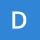Home
IT Knowledge
Inspiration
Languages
EN

# Java - iterate over array of strings

3 points
Created by:daniell
310

In this article, we would like to show you how to iterate over array of strings in Java.

Quick solution:

``````String[] letters = {"A", "B", "C"};

for (String letter : letters) {
System.out.println(letter);
}``````

## 1. Practical example using enhanced for loop

In this example, we use enhanced for loop to iterate over the `letters` array and print each element.

``````public class Example {

public static void main(String[] args) {
String[] letters = {"A", "B", "C"};

for (String letter : letters) {
System.out.println(letter);
}
}
}``````

Output:

``````A
B
C``````

## 2. Practical example using for loop

In this example, we use simple for loop to iterate over the `letters` array. This solution is equivalent to the above one, however we recommend to use enhanced for loop for its simplicity.

``````public class Example {

public static void main(String[] args) {
String[] letters = {"A", "B", "C"};

for (int i = 0; i < letters.length; i++) {
System.out.println(letters[i]);
}
}
}``````

Output:

``````A
B
C``````

## 3. Java 8 and streams

``````import java.util.Arrays;

public class Example {

public static void main(String[] args) {
String[] letters = {"A", "B", "C"};

// forEach + lambda
Arrays.stream(letters).forEach(s -> System.out.println(s));

// forEach + method reference
Arrays.stream(letters).forEach(System.out::println);
}
}``````

1. Java - for-each, for and forEach performance test